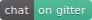# GGally: Extension to ggplot2ggplot2 is a plotting system for R based on the grammar of graphics. GGally extends ggplot2 by adding several functions to reduce the complexity of combining geoms with transformed data. Some of these functions include a pairwise plot matrix, a scatterplot plot matrix, a parallel coordinates plot, a survival plot, and several functions to plot networks.

## Installation

To install this package from Github or CRAN, do the following from the R console:

``````# Github
library(devtools)
install_github("ggobi/ggally")``````
``````# CRAN
install.packages("GGally")``````